# 4.3 Tight-Binding HAMILTONian

The general form of the tight-binding HAMILTONian for electrons in a CNT can be written as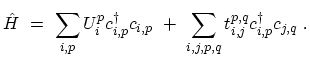(4.13)

The sum is taken over all rings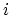,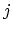along the transport direction, which is assumed to be the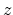-direction of the cylindrical coordinate system, and over all atomic locations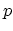,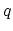in a ring. We use a nearest-neighbor tight-binding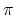-bond model [243,10]. Each atom in an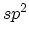-coordinated CNT has three nearest neighbors, located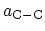away. The band-structure consists of-orbitals only, with the hopping parameter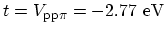and zero on-site potential. Furthermore, it is assumed that the electrostatic potential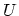rigidly shifts the on-site potentials. Such a tight-binding model is adequate to model transport properties in un-deformed CNTs.

In this work we consider zigzag CNTs. However, this method can be readily extended to armchair or chiral CNTs. Within the nearest-neighbor approximation, only the following parameters are non zero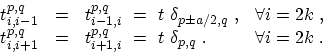(4.14)

Figure 4.6 shows that a zigzag CNT is composed of rings (layers) of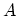- and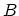-type carbon atoms, whereandrepresent the two carbon atoms in a unit cell of graphene. Each A-type ring is adjacent to a B-type ring. Within nearest-neighbor tight-binding approximation the total HAMILTONian matrix is block tri-diagonal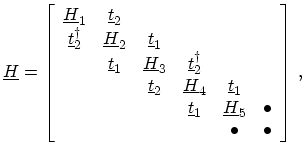(4.15)

where the diagonal blocks,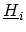, describe the coupling within an A-type or B-type carbon ring and off-diagonal blocks,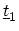and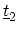, describe the coupling between adjacent rings. It should be noted that the odd numbered HAMILTONianrefer to A-type rings and the even numbered one to B-type rings. Each A-type ring couples to the next B-type ring according toand to the previous B-type ring according to. Each B-type ring couples to the next A-type ring according toand to the previous A-type ring according to. In a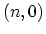zigzag CNT, there are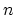carbon atoms in each ring, thus, all the sub-matrices in (4.15) have a size of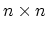.

In the nearest-neighbor tight binding approximation, carbon atoms within a ring are not coupled to each other so thatis a diagonal matrix. The value of a diagonal entry is the potential at that carbon atom site. In the case of a coaxially gated CNT, the potential is constant along the CNT circumference. As a result, the sub-matricesare given by the potential at the respective carbon ring times the identity matrix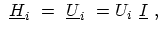(4.16)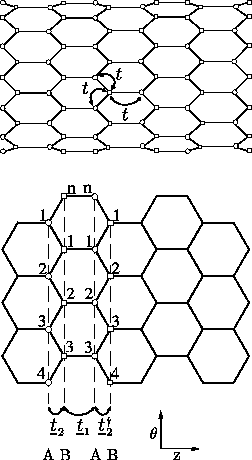There are two types of coupling matrices between nearest carbon rings,and. As shown in Fig. 4.6, the first type,, only couples an A(B) carbon atom to its B(A) counterpart in the neighboring ring. The coupling matrix is just the tight-binding coupling parameter times an identity matrix,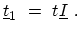(4.17)

The second type of coupling matrix,, couples an A(B) atom to two B(A) neighbors in the adjacent ring. The coupling matrix is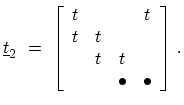(4.18)

The period of the zigzag CNT in the longitudinal direction contains four rings,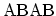, and has a length of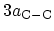. Therefore, the average distance between the rings is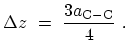(4.19)

M. Pourfath: Numerical Study of Quantum Transport in Carbon Nanotube-Based Transistors